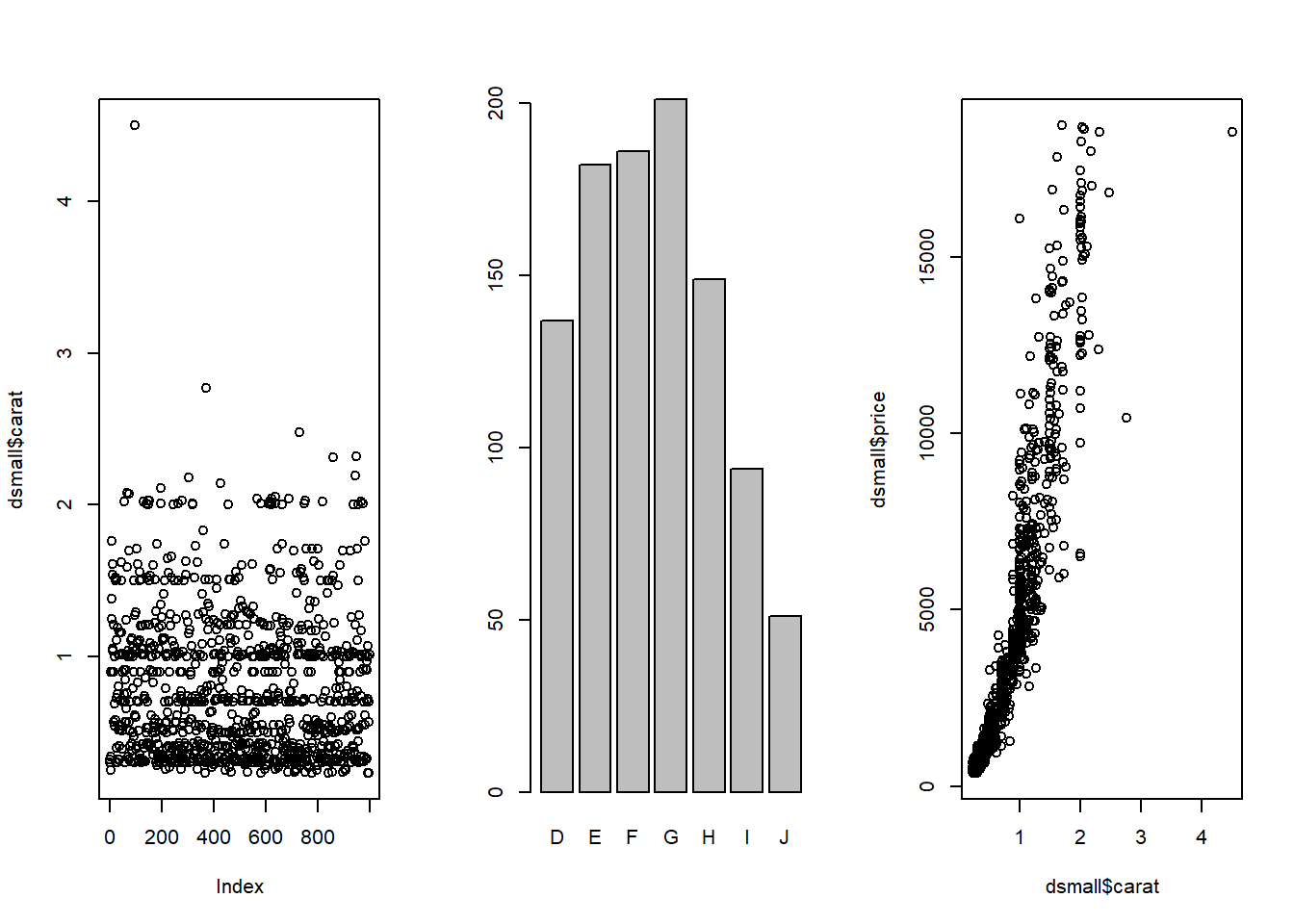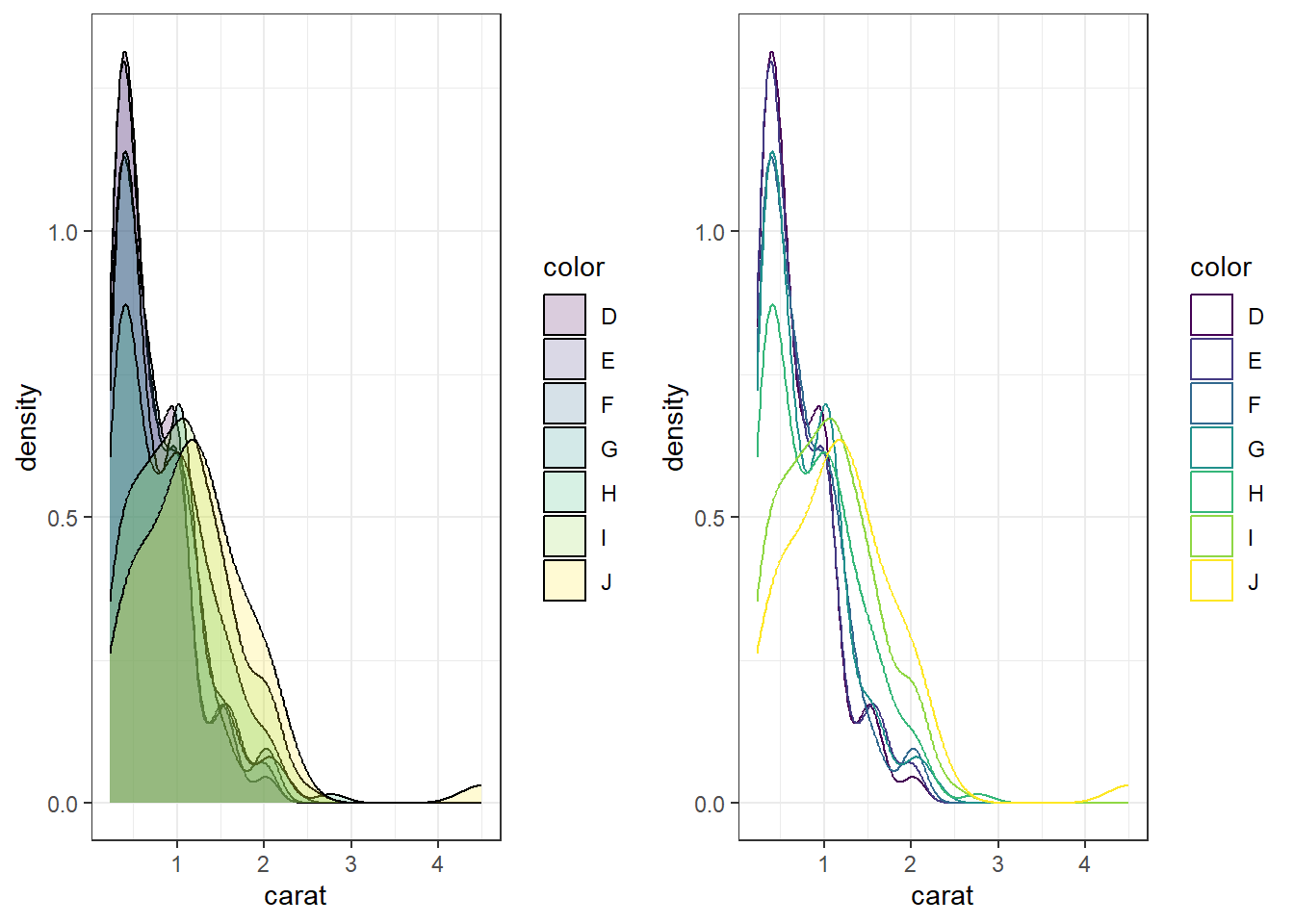## 2.6 Multiple plots per window

base I use par(mfrow=c(r,c)) for base graphics, where r is the number of rows and c the number of columns.

par(mfrow=c(1,3))
plot(dsmall$carat) plot(dsmall$color)
plot(dsmall$price ~ dsmall$carat)Other resources including learning about layouts. Multipanel plotting with base graphics http://seananderson.ca/courses/11-multipanel/multipanel.pdf

ggplot Use the grid.arrange function in the gridExtra package. I’ve done it several times above. You assign the output of a ggplot object to an object (here it’s plot1 and plot2). Then you use grid.arrange() to arrange them either side by side or top and bottom.

a <- ggplot(dsmall, aes(x=carat, fill=color)) + geom_density(alpha=.2)
b <- ggplot(dsmall, aes(x=carat, col=color)) + geom_density()
grid.arrange(a,b, ncol=2)